# Tune Control Systems in Simulink

This example shows how to use `systune` or `looptune` to automatically tune control systems modeled in Simulink.

### Engine Speed Control

For this example we use the following model of an engine speed control system:

```open_system('rct_engine_speed') ```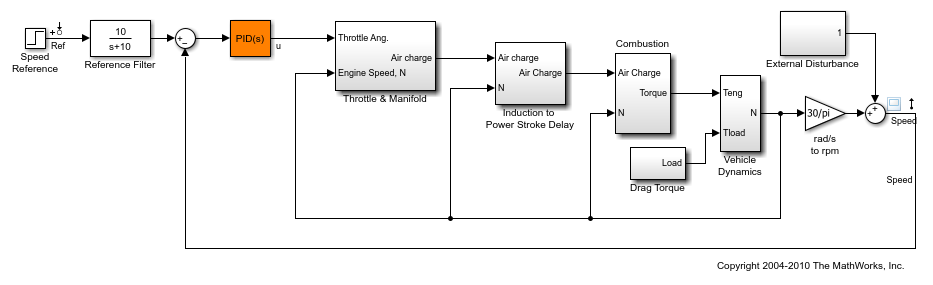The control system consists of a single PID loop and the PID controller gains must be tuned to adequately respond to step changes in the desired speed. Specifically, we want the response to settle in less than 5 seconds with little or no overshoot. While `pidtune` is a faster alternative for tuning a single PID controller, this simple example is well suited for an introduction to the `systune` and `looptune` workflows in Simulink.

### Controller Tuning with SYSTUNE

The `slTuner` interface provides a convenient gateway to `systune` for control systems modeled in Simulink. This interface lets you specify which blocks in the Simulink model are tunable and what signals are of interest for open- or closed-loop validation. Create an `slTuner` instance for the `rct_engine_speed` model and list the "PID Controller" block (highlighted in orange) as tunable. Note that all Linear Analysis points in the model (signals "Ref" and "Speed" here) are automatically available as points of interest for tuning.

```ST0 = slTuner('rct_engine_speed','PID Controller'); ```

The PID block is initialized with its value in the Simulink model, which you can access using `getBlockValue`. Note that the proportional and derivative gains are initialized to zero.

```getBlockValue(ST0,'PID Controller') ```
```ans = 1 Ki * --- s with Ki = 0.01 Name: PID_Controller Continuous-time I-only controller. ```

Next create a step tracking requirement to capture the target settling time of 5 seconds. Use the signal names in the Simulink model to refer to the reference and output signals, and specify the target response as a first-order response with time constant of 1 second.

```TrackReq = TuningGoal.StepTracking('Ref','Speed',1); ```

You can now tune the control system `ST0` subject to the requirement `TrackReq`.

```ST1 = systune(ST0,TrackReq); ```
```Final: Soft = 0.282, Hard = -Inf, Iterations = 65 ```

The final value is close to 1 indicating that the tracking requirement is met. `systune` returns a "tuned" version `ST1` of the control system described by `ST0`. Again use `getBlockValue` to access the tuned values of the PID gains:

```getBlockValue(ST1,'PID Controller') ```
```ans = 1 s Kp + Ki * --- + Kd * -------- s Tf*s+1 with Kp = 0.00217, Ki = 0.00341, Kd = 0.000513, Tf = 6.92e-07 Name: PID_Controller Continuous-time PIDF controller in parallel form. ```

To simulate the closed-loop response to a step command in speed, get the initial and tuned transfer functions from speed command "Ref" to "Speed" output and plot their step responses:

```T0 = getIOTransfer(ST0,'Ref','Speed'); T1 = getIOTransfer(ST1,'Ref','Speed'); step(T0,T1) legend('Initial','Tuned') ```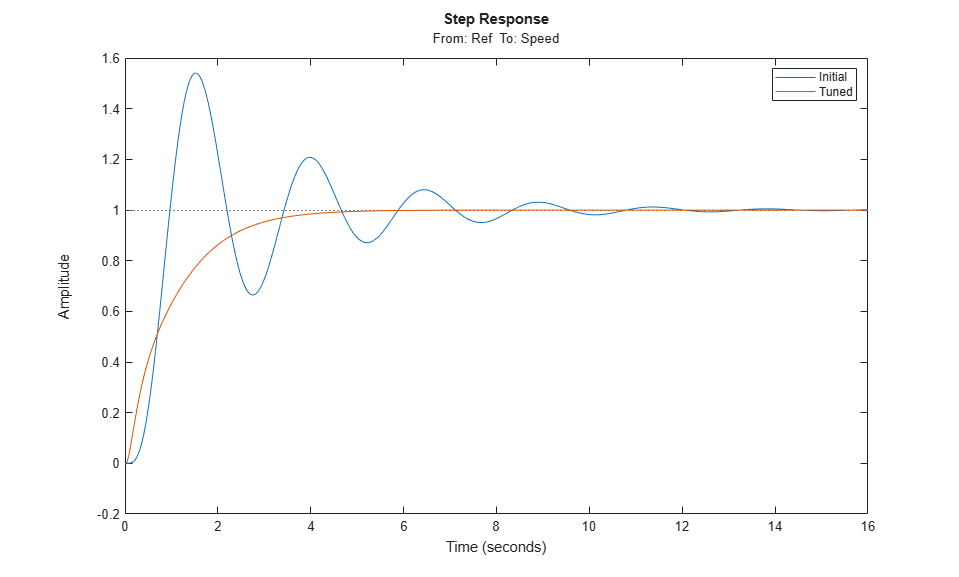### Controller Tuning with LOOPTUNE

You can also use `looptune` to tune control systems modeled in Simulink. The `looptune` workflow is very similar to the `systune` workflow. One difference is that `looptune` needs to know the boundary between the plant and controller, which is specified in terms of controls and measurements signals. For a single loop the performance is essentially captured by the response time, or equivalently by the open-loop crossover frequency. Based on first-order characteristics the crossover frequency should exceed 1 rad/s for the closed-loop response to settle in less than 5 seconds. You can therefore tune the PID loop using 1 rad/s as target 0-dB crossover frequency.

```% Mark the signal "u" as a point of interest addPoint(ST0,'u') % Tune the controller parameters Control = 'u'; Measurement = 'Speed'; wc = 1; ST1 = looptune(ST0,Control,Measurement,wc); ```
```Final: Peak gain = 0.979, Iterations = 4 Achieved target gain value TargetGain=1. ```

Again the final value is close to 1, indicating that the target control bandwidth was achieved. As with `systune`, use `getIOTransfer` to compute and plot the closed-loop response from speed command to actual speed. The result is very similar to that obtained with `systune`.

```T0 = getIOTransfer(ST0,'Ref','Speed'); T1 = getIOTransfer(ST1,'Ref','Speed'); step(T0,T1) legend('Initial','Tuned') ```You can also perform open-loop analysis, for example, compute the gain and phase margins at the plant input `u`.

```% Note: -1 because |margin| expects the negative-feedback loop transfer L = getLoopTransfer(ST1,'u',-1); margin(L), grid ```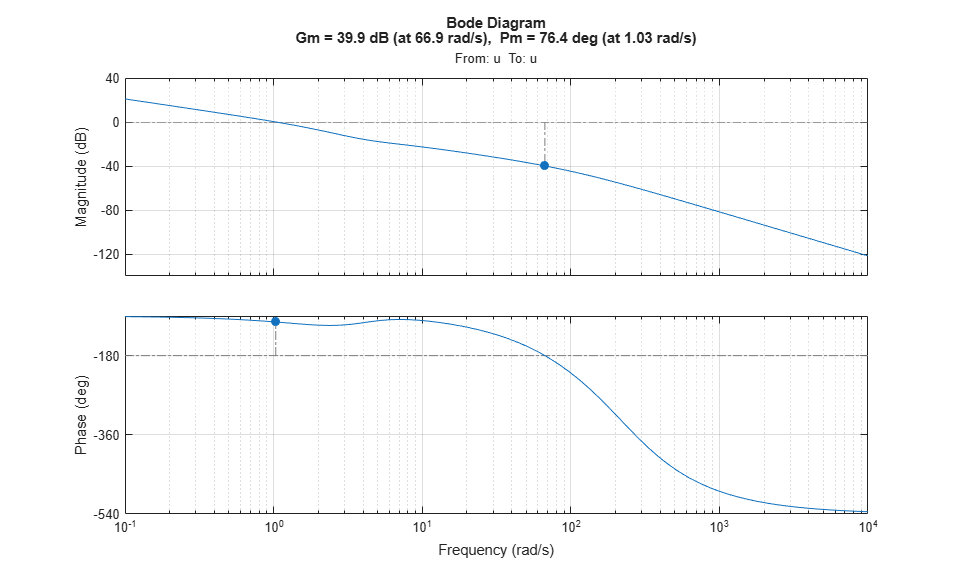Once you are satisfied with the `systune` or `looptune` results, you can upload the tuned controller parameters to Simulink for further validation with the nonlinear model.

```writeBlockValue(ST1) ```

You can now simulate the engine response with the tuned PID controller.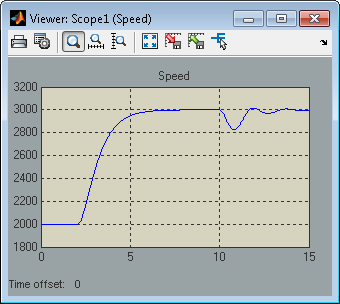The nonlinear simulation results closely match the linear responses obtained in MATLAB.

### Constraints on PID Gains

It is often useful to constrain the range of tuned parameters to weed out undesirable solutions. For example, you may require that the proportional and derivative gains of the PID controller be nonnegative. To do this, access the tuned block parameterization.

```C = getBlockParam(ST0,'PID Controller') ```
```C = Tunable continuous-time PID controller "PID_Controller" with formula: 1 s Kp + Ki * --- + Kd * -------- s Tf*s+1 and tunable parameters Kp, Ki, Kd, Tf. Type "pid(C)" to see the current value and "get(C)" to see all properties. ```

Set the "Minimum" value of the tunable parameters `Kp` and `Kd` to 0.

```C.Kp.Minimum = 0; C.Kd.Minimum = 0; ```

Finally, associate the modified parameterization with the tuned block.

```setBlockParam(ST0,'PID Controller',C) ```

Retune the PID gains and verify that the proportional and derivative gains are indeed nonnegative.

```ST1 = looptune(ST0,Control,Measurement,wc); showTunable(ST1) ```
```Final: Peak gain = 0.973, Iterations = 4 Achieved target gain value TargetGain=1. Block 1: rct_engine_speed/PID Controller = 1 s Kp + Ki * --- + Kd * -------- s Tf*s+1 with Kp = 0.000897, Ki = 0.00251, Kd = 0.000146, Tf = 0.01 Name: PID_Controller Continuous-time PIDF controller in parallel form. ```

### Comparison of PI and PID Controllers

Closer inspection of the tuned PID gains suggests that the contribution of the derivative term is minor. This suggests using a simpler PI controller instead. To do this, override the default parameterization for the "PID Controller" block:

```setBlockParam(ST0,'PID Controller',tunablePID('C','pi')) ```

This specifies that the "PID Controller" block should now be parameterized as a mere PI controller. Next re-tune the control system for this simpler controller:

```ST2 = looptune(ST0,Control,Measurement,wc); ```
```Final: Peak gain = 0.95, Iterations = 4 Achieved target gain value TargetGain=1. ```

Again the final value is less than one indicating success. Compare the closed-loop response with the previous ones:

```T2 = getIOTransfer(ST2,'Ref','Speed'); step(T0,T1,T2,'r--') legend('Initial','PID','PI') ```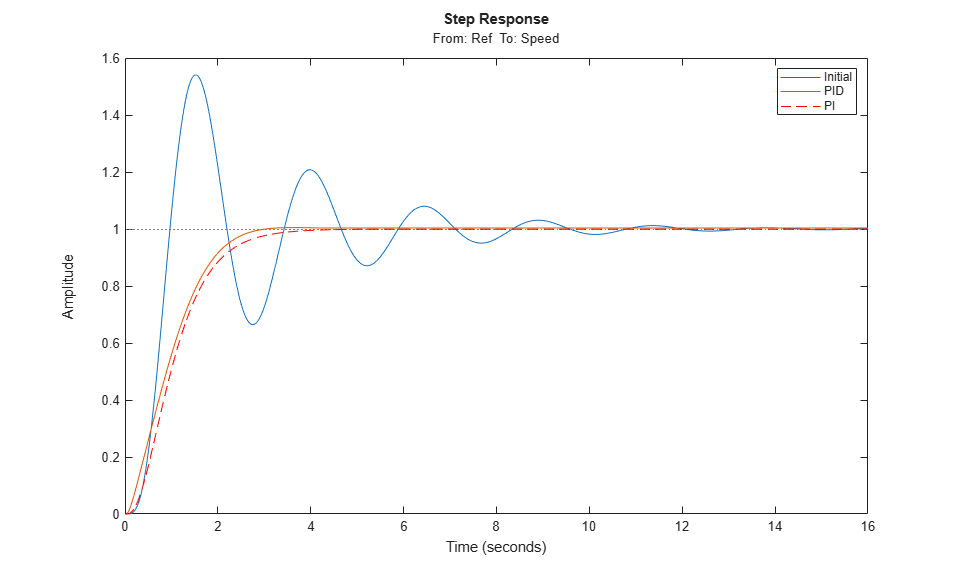Clearly a PI controller is sufficient for this application.